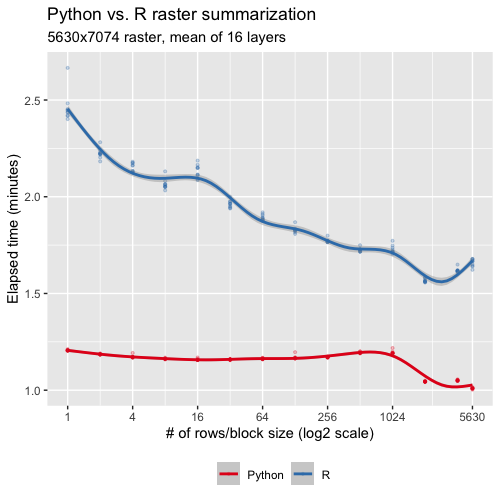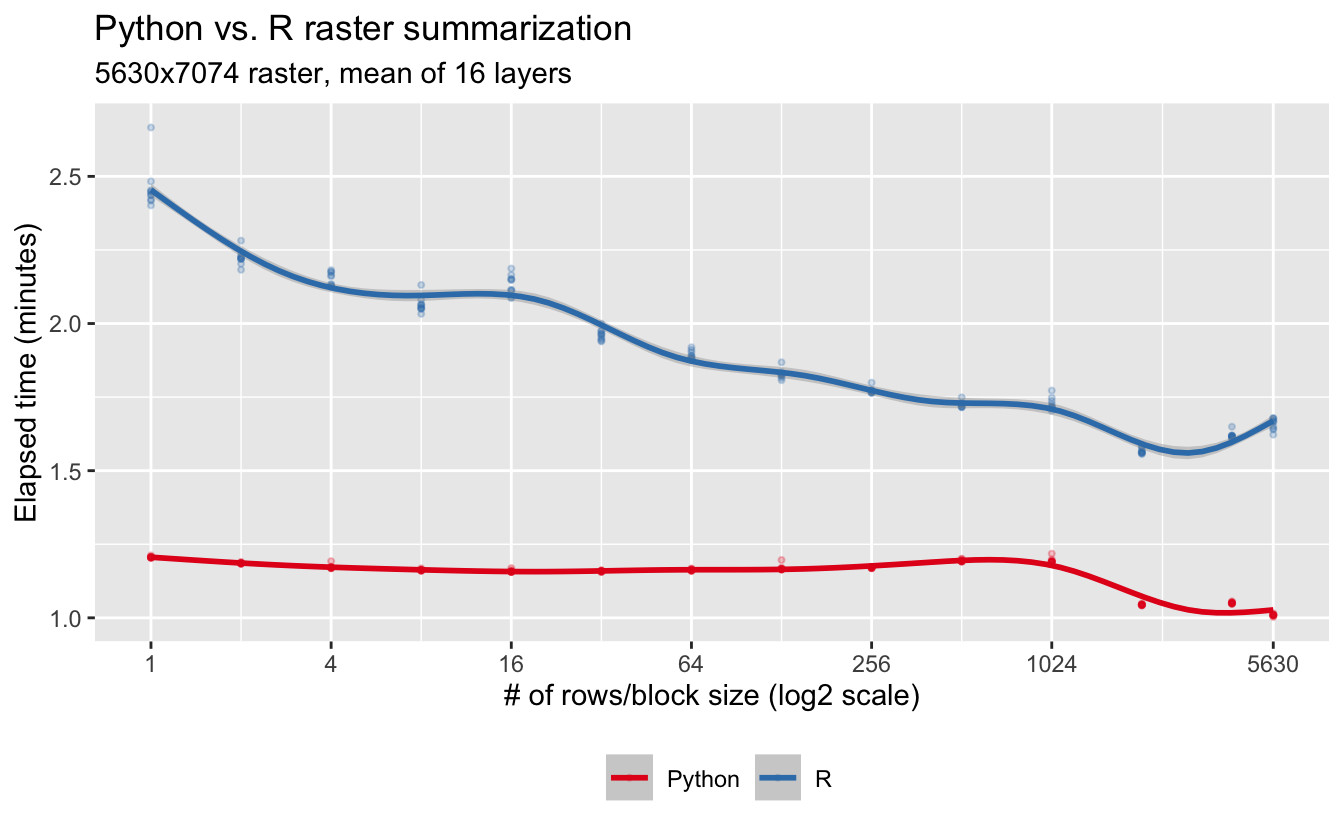# Raster Summarization in PythonAs part of the eBird Status & Trends team, I often find myself having to quickly summarize large rasters across layers. For examples, we might summarize weekly relative abundance layers for a single species across the weeks to estimate year round abundance or, for a given week, we might summarize across species to produce a species richness layer. I previously talked about how to perform these summarizations efficiently in R here and here. However, despite my best efforts, I could get the raster R package to perform as quickly as I wanted it to. After a little googling, I started to think maybe the answer to “how do I quickly summarize rasters across layers in R?” might be “screw it, use Python!”.

The gdal_calc.py tool is a general purpose raster calculator using GDAL. You can do all sorts of stuff with it: adding layers, multiplying layers, reclassification, and calculating the mean or sum, among many other things. I did a few tests, and it seemed to be faster than raster in R. There are three issues that made it a pain to work with though. First, you’re limited to working with a maximum of 26 layers or bands, and we often need to summarize across 52 weeks or hundreds of species. Second, in an effort to allow any possible type of raster algebra, the syntax is a bit verbose. For example, to find the cell-wise mean across two raster files, you’d use:

gdal_calc.py -A input.tif -B input2.tif --outfile=result.tif --calc="(A+B)/2"

which becomes cumbersome when you have many rasters. Finally, I found it hard to get gdal_calc.py to play nicely with missing values. I wanted to gdal_calc.py to ignore missing values similarly to mean(x, na.rm = TRUE) in R, but it wasn’t doing that.

To overcome these issues, I decided to teach myself Python and create my own tool, aided by the source code of gdal_calc.py, that’s specifically designed for summarizing raster data across layers or bands. This tool is gdal-summarize.py and is available on GitHub. I hope others will use it and provide feedback; this is my first foray into Python!

## gdal_summarize.py

The goal of gdal-summarize.py is to summarize raster data across layers or bands. There are two common use cases for this tool. The first is calculating a cell-wise summary across the bands of a raster file (e.g. a GeoTIFF). For example, given a multi-band input GeoTIFF file input.tif, to calculate the cell-wise sum of the first three bands use:

gdal-summarize.py input.tif --bands 1 2 3 --outfile output.tif

Alternatively, to compute the cell-wise sum across multiple GeoTIFF files (input1.tif, input2.tif, and input3.tif) use:

gdal-summarize.py input1.tif input2.tif input3.tif --outfile output.tif

If these input files have multiple bands, the default behavior is to summarize them across the first band of each file; however, the --bands argument can override this behavior:

# summarize across the second band of each file
gdal-summarize.py input1.tif input2.tif input3.tif --bands 2 --outfile output.tif
# summarize across band 1 of input1.tif and band 2 of input2.tif
gdal-summarize.py input1.tif input2.tif --bands 1 2 --outfile output.tif

### Summary functions

The default behavior is to perform a cell-wise sum; however, other summary functions are available via the --function argument:

• sum: cell-wise sum across layers.
• mean: cell-wise mean across layers.
• count: count the number layers with non-negative value for each cell.
• richness: count the number of layers with positive values for each cell.

In all cases, these functions remove missing values (i.e. NoData or NA) prior to calculating the summary statistic. For example, the mean of the numners, 1, 2, and NoData is 1.5. This is similar to the way na.rm = TRUE works in R. I’d be happy to add additional summary functions, just open an issue or submit a pull request on GitHub.

### Block processing

To avoid having to read an entire raster file into memory, gdal-summarize.py processes input rasters in blocks; it will read in a block of data, summarize it, then output to file, before proceeding to the next block. GDAL will suggest a “natural” block size for efficiently processing the data (typically 256X256 cells for GeoTIFFs), but users can also specify their own block size with command line arguments in one of two ways:

• --block_size/-s: two integers given the x and y dimensions of the block. Note that the x dimension corresponds to columns, while the y dimension corresponds to rows.
• --nrows/-n: an integer specifying the number of rows in each block. If this approach is used, the raster will be processed in groups of entire rows.

It’s not clear to me what the most efficient block size is, so using the default block size is probably a good starting point. This StackExchange question has some thoughts on block size and efficiency and suggests it could be worth doing some tests to see what works best for your scenario.

## Comparing Python and R

Everyone loves a good Python vs. R battle, so let’s put calc() from the raster R package up against gdal-summarize.py. I use the same Wood Thrush relative abundance raster from eBird Status and Trends as I used in my previous post, consisting of 16 bands with dimensions 5630X7074. Since raster processes data in blocks of rows, I’ll do the same thing for gdal-summarize.py.

library(raster)
library(tidyverse)

f <- "data/woothr.tif"
r <- stack(f)

# choose the values of n_rows to try
n_rows <- 2^seq(0, ceiling(log2(nrow(r))))
n_rows[length(n_rows)] <- nrow(r)

# set up 10 repetitions of each n_rows value
nrow_grid <- expand_grid(rep = 1:10, n_rows = n_rows)

# summarize raster using each value of n_rows
summarize_nrows <- function(x) {
# time r
t_r <- system.time({
r_mean <- summarize_raster(r, "mean", n_rows = x)
})
# time python
f_out <- "woothr-mean.tif"
t_py <- system.time({
str_glue("source ~/.bash_profile;",
"gdal-summarize.py {f} -o {f_out} -w -f 'mean' -n {x}") %>%
system()
})
tibble(elapsed_r = t_r["elapsed"], elapsed_py = t_py["elapsed"])
}
time_summarize <- nrow_grid %>%
mutate(results = map(n_rows, summarize_nrows)) %>%
unnest(cols = results)

Let’s take look at how they compare:

# x-axis breaks
brks <- 2^seq(0, ceiling(log2(nrow(r))), by = 2)
brks[length(brks)] <- nrow(r)

# transform to longer for ggplot
time_summarize <- time_summarize %>%
pivot_longer(cols = c(elapsed_r, elapsed_py)) %>%
mutate(name = recode(name, "elapsed_r" = "R", "elapsed_py" = "Python"))

# plot
ggplot(time_summarize) +
aes(x = n_rows, y = value / 60, group = name, color = name) +
geom_point(alpha = 0.25, size = 0.75) +
geom_smooth(method = "gam") +
scale_x_continuous(trans = "log2", breaks = brks) +
scale_color_brewer(palette = "Set1") +
labs(x = "# of rows/block size (log2 scale)",
y = "Elapsed time (minutes)",
color = NULL,
title = "Python vs. R raster summarization",
subtitle = "5630x7074 raster, mean of 16 layers") +
theme(legend.position = "bottom")So, not only is Python raster than R, but Python experiences much less of a block size effect.

Hopefully others will find this tool useful! Please take a look through the GitHub repo and let me know if there’s something I could be doing differently. As I said at the start, this is my first foray into Python, so I’m bound to have made some mistakes.##### Matt Strimas-Mackey
###### Data Scientist

I am a data scientist at the Cornell Lab of Ornithology using data from the citizen science project eBird to inform bird research and conservation.